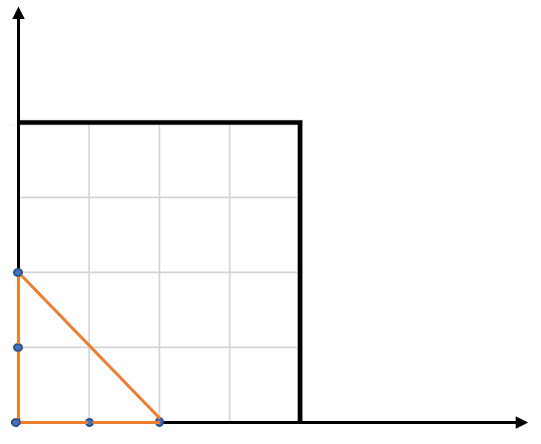# Leetcode 812 - Largest Triangle Area

Note:

• Know how to use cross product to calculate area.
• $Area = |AB x AC| = |a_xby - a_y*b_x|$
• Because $|AB x AC| = |AB||AC|sin_{\theta}$, it’s the area of the parallelogram. Divide it by 2, it’s the area of the triangle!

Question:

Given an array of points on the X-Y plane points where points[i] = [xi, yi], return the area of the largest triangle that can be formed by any three different points. Answers within $10^{-5}$ of the actual answer will be accepted.

Example:Code: